• 析取范式合取范式、主析取和主合取
万次阅读
2020-04-20 16:55:40

了解一下简单析取式和简单合取式，简单析取式说白了就是有限个命题变项或者他们的否定用符号v连接起来的式子，比如p,¬p,pvq,pv¬q等，简单合取式类似，但它用的符号是Λ。析取范式简单来说就是几个简单析取式的组合，合取范式类似。

而主合取和主析取略微有些不同，主析取是由极小项及符号v组成，极小项就是简单合取式，说白了主析取就是由符号v连接几个简单合取式组成，且他的每一个构成单位（极小项）的命题变项的个数都是一样的，举个例子：(pΛq)v(qΛr)，这不是主析取，因为这个式子总共有三个命题变项，p,q,r,第一个单元pΛq并未包含r，而第二个式子qΛr里也没有p，不完整，不能成为主析取范式，这个时候我们想要让他成为主析取范式则要进行操作，对第一个式子加上缺少的项r，怎么加呢？答案是将第一个式子用(pΛq)Λ(rv¬r)代替，因为极小项对应成真赋值交上一个rv¬r原式并未改变，然后合理运用分配律变换式子，使其变成主析取范式。主合取刚好相反，主合取对应极大项，成假赋值，对应如下：

主析取–极小项（简单合取式）–成真赋值（式子Λ（xv¬x））
析——小——真
主合取–极大项（简单析取式）–成假赋值（式子v(xΛ¬x）)
合——大——假

析取范式变成主析取的时候结果出现的项对应的角码即其成真赋值，未出现的为成假赋值，合取范式变成主合取的结果出现的项对应的角码即其成假赋值，未出现的是成真赋值。（n个项对应2的n次方种情况，其二进制角码转换成十进制数字即下标，如000对应m。，001对应m1等）

更多相关内容
• 2.2析取范式与合取范式 本节给出命题公式的两种规范表示方法,这种规范的表达式能表达真值表所能提供的一切信息 定义2.2命题变项及其否定统称作文宇.仅由有限个文字构成的析取式称作简单析取式仅由有限个文字构成...

2.2析取范式与合取范式

本节给出命题公式的两种规范表示方法,这种规范的表达式能表达真值表所能提供的一切信息

定义2.2命题变项及其否定统称作文宇.仅由有限个文字构成的析取式称作简单析取式仅由有限个文字构成的合取式称作简单合取式

P,┓g;pV┓p,┓pVq和┓pV┓qVr,pV┓pVr都是简单析取式,分别由1个文字,2个文字和3个文字构成

┓p,q;p^p,P^┓q和p∧q^┓r, ┓P^P^q都是简单合取式,分别由1个文字,2个文字和3个文字构成.注意,一个文字既是简单析取式、又是简单合取式

设A是含n个文字的简单析取式,若Ai中既含某个命题变项Pj又含它的否定式┓Pj,由交换律、排中律和零律可知,Ai为重言式.反之,若Ai为重言式,则它必同时含某个命题变项及它的否定式.否则若将A,中的不带否定符的命题变项都取0值,带否定符的命题变项都取1值,此赋值为Ai的成假赋值,这与Ai是重言式相矛盾.类似地,设Ai是含n个命题变项的简单合取式若Ai中既含某个命题变项Pj又含它的否定式┓Pj则Ai,为矛盾式.反之,若Ai为矛盾式,则Ai中必同时含某个命题变项pj及它的否定式.于是,得到下面的定理

定理2.1 (1)简单析取式是重言式当且仅当它同时含某个命题变项及它的否定式

1. 一个简单合取式是矛盾式当且仅当它同时含某个命题变项及它的否定式

定义2.3由有限个简单合取式的析取构成的命题公式称为析取范式,由有限个简单析取式的合取构成的命题公式称为合取范式,

析取范式与合取范式统称为范式析取范式的一般形式为A1VA2V…VAi,其中A,(i=1,2,…,s)为简单合取式;合取范式的般形式为B1^B2^...^Bj,其中B(j=1,2,…,t)为简单析取式例如,(P^┓q)V(┓q^┓r)Vp为析取范式(pVqVr)^(┓pV┓q)^(┓pV┓rVs)为合取范式.┓P^q^r既是由一个简单合取式构成的析取范式,又是由3个简单析取式构成的合取范式;类似地, pv┓qvr既是由3个简单合取式构成的析取范式,又是由一个简单析取式构成的合取范式。(看定义)

析取范式和合取范式具有下述性质

定理2.2 (1)一个析取范式是矛盾式当且仅当它的每个简单合取式都是矛盾式

(2)一个合取范式是重言式当且仅当它的每个简单析取式都是重言式

到现在为止,我们研究的命题公式中含有5个联结词{∧,V,┓,→,<->},如何把这样的命题公式化成等值的析取范式和合取范式？

首先,可以利用蕴涵等值式与等价等值式

A→B<=>┓AVB |

A<->B<=>(┓AVB)∧(AV┓B) |(2.17)

消去任何公式中的联结词→和<->

其次,在范式中不出现如下形式

┓┓A, (┓A^B), ┓(AVB)

对其利用双重否定律和德摩根律,可得

┓┓A<=>A |

┓(A^B)<=>┓AV┓B |

┓(AVB)<=>┓A^┓B |(2.18)

再次,在析取范式中不出现如下形式:

A^(BVC)

在合取范式中不出现如下形式

AV(B^C)

利用分配律,可得

A^(BVC)<=>(A^B)V(A^C)

AV(B^C)<=>(AVB)^(AVC)

上述3步,可将任一公式化成与之等值的析取范式和合取范式.于是,得到下述定理

定理2.3(范式存在定理) 任一命题公式都存在与之等值的析取范式与合取范式

求给定公式范式的步骤

1. 为消去联结词->,<=>
2. 用双重否定律消去双重否定符,用德摩根律内移否定符3.
3. 使用分配律:求析取范式时使用^对V的分配律,求合取范式时使用V对^的分配律

例2.7求下面公式的析取范式与合取范式:

(p->q)<->r

解为了清晰和无误,利用交换律使每个简单析取式和简单合取式中命题变项都按字典顺出现。

1. 先求合取范式

(p->q)<->r

<=> (┓pVq)<->r (消去→)

<=> ((┓pVq)→r)∧(r→(┓pVq)) (消去<->)

<=> (┓(┓pVq)Vr)∧(┓rV┓pVq) (消去→)

<=> ((P∧┓q)Vr)∧(┓pVqV┓r) (否定符内移)台

<=> (PVr)∧(qVr)∧(┓pVq┓r) (v对∧的分配律)

含3个简单析取式的合取范式

1. 求析取范式求析取范式与求合取范式的前两步是相同的,只是在利用分配律时有所不同,因而前4步与(1)相同,接着使用∧对V的分配律

(p-q)<->r

<=>((p^┓q)Vr)^( ┓pVqV┓r)

<=>(p^┓q^┓p)V(P^┓q^q)V(p^┓q^┓r)

V(r^q)V(r^┓q) (^对V的分配律)

<=>(p^┓q^┓r)V(┓p^r)V(q^r) (矛盾律和同一律)

最后两步的结果都是析取范式,一般地,命题公式的析取范式是不惟一的同样,合取范式也是不惟一的为了使命题公式的范式惟一,进一步将简单合取式和简单析取式规范化,定义如下

定义2.4在含有n个命题变项的简单合取式(简单析取式)中,若每个命题变项和它的否定式恰好出现一个且仅出现一次,而且命题变项或它的否定式按下标从小到大或按字典顺序排列,称这样的简单合取式(简单析取式)为极小项(极大项)

由于每个命题变项在极小项中以原形或否定形式出现且仅出现一次,因而n个命题变项共可产生2"个不同的极小项.每个极小项都有且仅有一个成真赋值.若极小项的成真赋值所对应内二进制数等于十进制数i,就将这个极小项记作mi类似地,n个命题变项共可产生2"个不同的极大项,每个极大项只有一个成假赋值,将其对应的十进制数i做极大项的角标,记作Mi 表2.3和表2.4分别列出含p,q与p,q,r的全部极小项和极大项

根据表2.3和表2.4可以直接验证极小项与极大项之间有下述关系

定理2.4设mi与Mi是命题变项含P1,P2,...,P3的极小项和极大项,则

┓mi<=Mi ┓Mi<=>mi

定义2.5所有简单合取式(简单析取式)都是极小项(极大项)的析取范式(合取范式)为主析取范式(主合取范式)

下面讨论如何求出与给定公式等值的主析取范式和主合取范式,首先证明它的存在性和惟一性,再给出它的求法

定理2.5任何命题公式都存在与之等值的主析取范式和主合取范式,并且是惟一的

这里只证主析取范式的存在性和惟一性

首先证明存在性设A是任一含n个命题变项的公式.由定理2.3可知,存在与A等值的析取范式A',即A<=>A’·若A'的某个简单合取式A,中既不含命题变项Pj,也不含它的否定式┓Pj,则将Ai展成如下等值的形式

A1^(PjV┓pj)<=>(Ai^Pj)V(Ai^┓Pj)继续这个过程,直到所有的简单合取式都含有所有的命题变项或它的否定式

若在演算过程中出现重复出现的命题变项以及极小项和矛盾式时,都应“消去”:如用p代替p∧p,m代替miVmi,0代替矛盾式等.最后就将A化成与之等值的主析取范式A"

下面再证明惟一性.假设命题公式A等值于两个不同的主析取范式B和C,那么必有B<=>C由于B和C是不同的主析取范式,不妨设极小项mi只出现在B中而不出现在C中.于是,角标i的二进制表示为B的成真赋值,而为C的成假赋值,这与B<=>C矛盾

主合取范式的存在惟一性可类似证明

在证明定理2.5的过程中,已经给出了求主析取范式的步骤为了醒目和便于记忆,求出某公式的主析取范式(主合取范式)后,将极小项(极大项)都用名称写出,并且按极小项(极大项)名称的角标由小到大顺序排列

例2.8求例2.7中公式的主析取范式和主合取范式

解(1)求主析取范式在

例2.7中已给出公式的析取范式,即

(p-q)<->r

<=>(p^┓q^┓r)V(┓p^r)V(q^r)

在此析取范式中,第一项p^┓q^┓r是极小项m4。,另外两个简单合取式┓p^r,q^r都不是极小项.下面先分别求出它们派生的极小项,注意,因为公式含有3个命题变项,所以极小项均由3个文字组成

┓p^r

<=> ┓p^(┓qVq)^r

<=> (┓p^┓q^r)V(┓p^qVr)

<=> m1Vm3

Q^r

<=>(┓pVp)^q^r

<=>(┓p^q^r)V(p^q^r)

<=>m3Vm7

于是

(p->q)<->r<=>m1Vm3Vm4Vm7

(2)求主合取范式

由例2.7已求出公式的合取范式，

(p->q)<->r

<=> (pVr)^(┓qVr)^(┓pVqV┓r)

其中┓pVqV┓r已是极大项M5，利用矛盾律和同一律将另两人个简单析取式化成极大项

Pvr

<=>pV(q^┓q)Vr

<=>(pVqVr)^(pV┓qVr)

<=>M0Vm2

┓qVr

<=>(p^┓p)V┓qVr)

<=>(pV┓qVr)^(┓pV┓qVr)

<=>M2Vm6

于是

(p->q)<->r<=>M0VM2VM3VM6

例2.9 求命题公式p->q的主析取范式与主合取范式。

解 本公式 中含两人个命题变项，所心权小项和极大项均含两个文字。

1. P->q

<=>┓pVq

<=>M2 (主合取范式)

1. P->q

<=>┓pVq

<=>(┓p^(┓qVq))V((┓pVp)^q)

<=>(┓p^┓q)V(┓p^q)V(┓p^q)V(p^q)

<=>(┓p^┓q)V(┓p^q)V(p^q)

<=>m0Vm1Vm3 (主析取范式)

由例2.8与2.9可知,在求给定公式的主析取范式(主合取范式)时,一定要根据公式中命题变项的个数决定极小项(极大项)中文字的个数.

下面讨论主析取范式的用途(主合取范式可类似讨论).主析取范式像真值表一样,可以表达出公式以及公式之间关系的一切信息

1. 求公式的成真赋值与成假赋值

若公式A中含n个命题变项,A的主析取范式含s(0≤s≤2n)个极小项,则A有s个成真赋值,它们是所含极小项角标的二进制表示,其余2n-s个赋值都是成假赋值.例如,例2.8中(p→q)<->r<=>m1Vm3Vm4Vm7.这里有3个命题变项,将主析取范式中各极小项的角标1,3,4,7写成长为3的二进制数,它们分别为001,011,100,111这4个赋值即为该公式的成真赋值.而主析取范式中未出现的极小项m0,m2,m3,m6。的角标的二进制表示000,010,101,110为该公式的成假赋值.又如例2.9中,p→q<=>m0Vm,Vm3,含两个命题变项,极小项的角标的二进制表示00,01,11为该公式的成真赋值,而10是它的成假赋值

1. 判断公式的类型

设公式A中含n个命题变项,容易看出

1. A为重言式当且仅当A的主析取范式含全部2°个极小项
2. A为矛盾式当且仅当A的主析取范式不含任何极小项.此时,记A的主式为0
3. A为可满足式当且仅当A的主析取范式中至少含一个极小项

例2.10用公式的主析取范式判断下述公式的类型

1. ┓(p->q)^q
2. p→(PVq)
3. (pVg)→r

注意,(1),(2)中公式含两个命题变项,极小项含两个文字,而(3)中公式含3个命题变项,因而极小项中应含3个文字

1.  ┓(p->q)^q

<=> ┓(┓pVq)^q

<=> (p^┓q)^q

<=>0

这说明该公式是矛盾式。

1. P->(pVq)

<=>┓pVpVq

<=>(┓p^(┓qVq))V(p^(┓qVq))V((┓pVp)^q)

<=>(┓p^┓q)V(┓p^q)V(p^┓q)V(p^q)V(┓p^q)V(p^q)

<=>(┓p^┓q)V(┓p^q)V(p^┓q)V(p^q)

<=>m0Vm1Vm2Vm3

由于主析取范式含两个命题变项的全部22=4个极小项，故该公式为重言式。

其实,以上演算到第就已知该公式等值于1,因而它为重言式,如果要写出它的主析取范式,由1可直接写出全部极小项：

1. >(pVq)

<=>┓pVpVq

<=>1

<=>m0Vm1Vm2Vm3

1. (pVq)->r

<=>┓(pVq)Vr

<=>(┓p^┓q)Vr

<=>(┓p^┓q)Vr(下为凑式子）

<=>(┓p^┓q^(┓rVr))V((┓pVp)^(┓qVq)^r)

<=>(┓p^┓q^┓r)V(┓p^┓q^r)V(┓p^q^r)v(p^┓

<=>(┓p^┓q^┓r)V(┓p^┓q^r)V(┓p^q^r)V(p^┓q^r)V(p^q^r)

<=>m0Vm1Vm3Vm5Vm7

该公式是可满足的，但不是重言式，因为它的主析取范式没含全部8个极小项。

1. 判断两个命题公式是否等值
1. 设公式A,B共含有n个命题变项，按n个命题变项求出A与B=B’,则A<=>B，否则A<≠>B

例2.11判断下面两组公式是否等值

1. P与(P^q)V(p^┓q)
2. (P→q)→r与(P^q)->r

(1)这里有2个命题变项,因而极小项含2个文字

P

<=>p^(┓qVq)

<=>(p^┓q)V(p^q)

<=>m2 Vm3

(p^q)V(p^┓q)

<=>m2,Vm3

所以

P<=>(p^┓q)V(p^q)

这里有3个命题变项,因而极小项含3个文字.经过演算得到

(p->q)->r

<=>m1Vm3Vm4Vm5Vm7

(p^q)->r

<=>m0Vm1Vm2Vm3Vm4Vm5Vm7

两者的主析取范式不同，所以

(p->q)->r <≠> (p^q)->r

最后举一个应用主析取范式分析和解决实际问题的例子

例2.12某科研所要从3名科研骨干A,B,C中挑选1~2名出国进修.由于工作需要，选派时要满足以下条件：

1. 若A去,则C同去.
2. 若B去,则C不能去
3. 若C不去,则A或B可以去

问所里有哪些选派方案?

解 设p:派A去

q:派B去

R:派C去

由已知条件可得公式(p->r)^(q->┓r)^(┓r->(pvq))

该公式的成真赋值即为可行的选派方案.经过演算得到

(p->r)^(q->┓r)^(┓r->(pvq))

<=>(┓pVr)^(┓qV┓r)^(┓┓rV(pVq))

<=>((┓pVr)^┓q)V((┓pVr)^┓r)^(pVqVr)

<=>((┓p^┓q)V(r^┓q))V((┓p^┓r)V(r^┓r))^(pVqVr)

<=>(┓p^┓q^p)V(p^┓q^r)V...(此处展开大量计算略)

<=>(┓p^┓q^r)V(┓p^q^┓r)V(p^┓q^r)

<=>m1, V m2, V m3故有3种选派方案

1. C去,A,B都不去
2. B去,A,C都不去
3. A,C同去,B不去

以上讨论了主析取范式的求法与用途,也可对主合取范式做类似的讨论.关于主合取范式还要说明以下两点

1. 由主析取范式求主合取范式

设公式A含n个命题变项,A的主析取范式含s(0<s<2n)个极小项,即

A=mi1Vmi2...Vmin, 0<ij<2n-1,j=1,2,...s.

没出现的极小项为mj1,mj2,...,mj2n-1它们的角标的二进制表示为┓A的成真赋值,因而┓A的主析取范式为

┓A=mj1Vmj2V...Vmj2n-1

这就由公式的主析取范式直接求出它的主合取范式

例2.13利用公式的主析取范式,求主合取范式:

1. A<=>m1Vm2(A中含2个命题变项p,q)
2. B<=>m1Vm2Vm3(B中含3个命题变项p,q,r)

(1)由题可知,没出现在主析取范式中的极小项为m0和m3,所以A的主合取范式中含两个极大项M0与M3,故

A<=>M0^M3

(2)B的主析取范式中没出现的极小项为mo,m4,m5,m6,m7因而

B<=>M0^M4^M5^M6^M7

反之,也可由公式的主合取范式给出主析取范式

1. 重言式与矛盾式的主合取范式

矛盾式无成真赋值,因而矛盾式的主合取范式含全部2n(n为公式中命题变项个数)个极大项.而重言式无成假赋值,主合取范式不含任何极大项,规定重言式的主合取范式为1.至于可满足式,它的主合取范式中极大项的个数一定小于2n

最后,要问:n个命题变项的主析取范式(主合取范式)共有多少个?n个命题变项共可产生个极小项(极大项),因而共可产生22n

个不同的主析取范式(主合取范式).这与在1.2节中对真值表个数的讨论情况是一样的

事实上,A<=>B当且仅当A与B有相同的真值表,又当且仅当A与B有相同的主析取范式(主合取范式).因而可以说,真值表与主析取范式(主合取范式)是描述命题公式的两种等价的不同标准形式,两者可以相互确定,由A的主析取范式(主合取范式)可以立刻确定A的真值表由A的真值表也可以立刻确定A的主析取范式(主合取范式).

展开全文离散数学 数理逻辑
• 本资料为原书第2版PDF转为WORD形式而成，略有补充，仅供学习参考！
• 1.4 析取范式与合取范式 这一小节内容较多，我们由浅入深的来。首先要明白简单析取式和简单合取式的定义。 定义：我们将命题变项及其否定统称作文字\red{文字}文字。 简单析取式\red{简单析取式}简单析取式是仅...

# 1.4 析取范式与合取范式

这一小节内容较多，我们由浅入深的来。首先要明白简单析取式和简单合取式的定义。

## 例如：

• p ， ¬q既是一个简单析取式，又是一个简单合取式
• p ∨ \vee ¬q , p ∨ \vee r 均是有两个文字的简单析取式
• p ∧ \wedge q ∧ \wedge r , ¬ p ∧ \wedge q ∧ \wedge ¬q 均是有三个文字的简单合取式

## 性质：

1. 一个简单析取式是重言式当且仅当它同时含某个命题变项及它的否定式。
2. 一个简单合取式是矛盾式当且仅当它同时含某个命题变项及它的否定式。

## 3. 析取范式与合取范式统称为 范 式 \red{范式}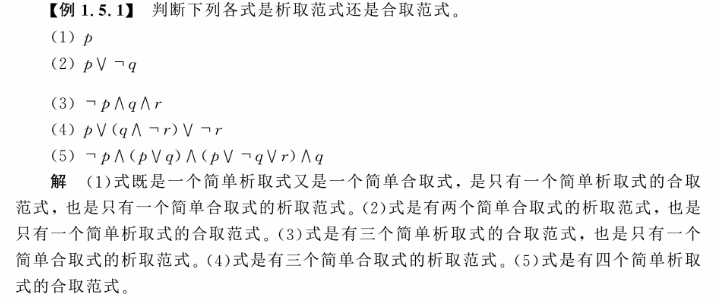## 性质：

1. 一个文字既是一析取范式又是一合取范式
2. 一个析取范式为矛盾式，当且仅当它的每个简单合取式都是矛盾式
3. 一个合取范式是重言式当且仅当它的每一个简单析取式都是重言式

## 范式存在定理：任一命题公式都存在着与之等值的析取范式与合取范式。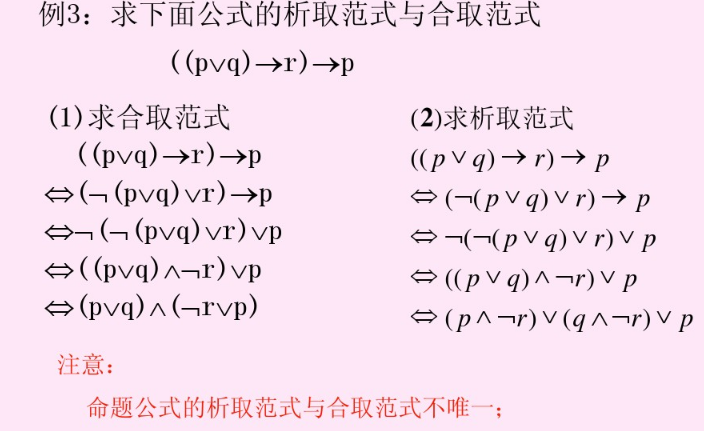范式，顾名思义即规范的式子。我们想找到对某一命题公式有唯一规范的式子，显然析取范式和合取范式还不能达到我们的目的。所以我们又在此基础上对析取范式和合取范式加一些限制，使得他们的形式是唯一的。即引申出主析取范式和主合取范式。而主析取范式和主合取范式分别是由极小项和极大项组成的。

## 极大项：包含公式A中 每 一 个 \red{每一个} 命题变元或其否定 一 次 且 仅 一 次 \red{一次且仅一次} 的简单析取式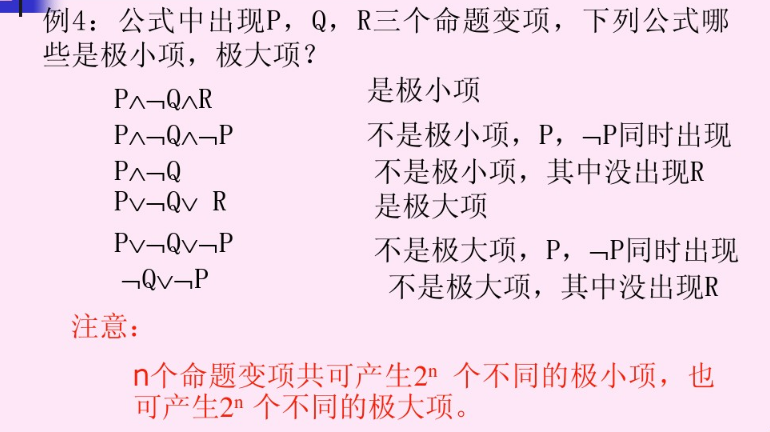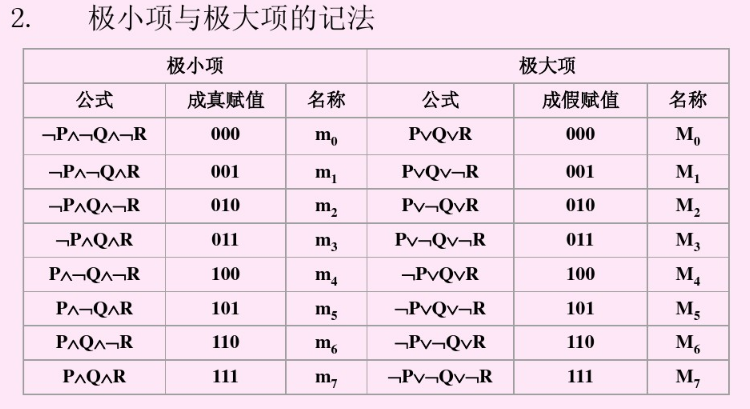## 定理：任何命题公式的主析取范式（主合取范式）都是存在的，并且是 唯 一 \red{唯一} 的

对此定理的证明也是主析取范式（主合取范式）的求解过程，如下：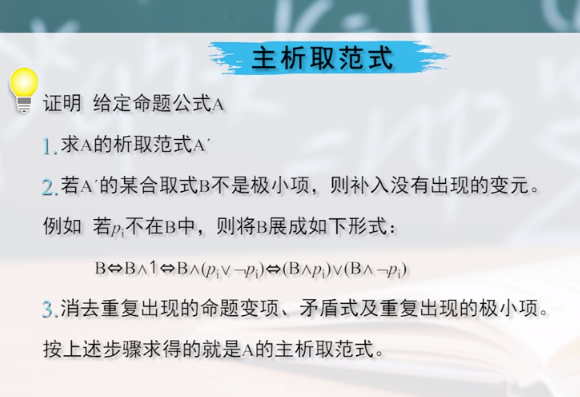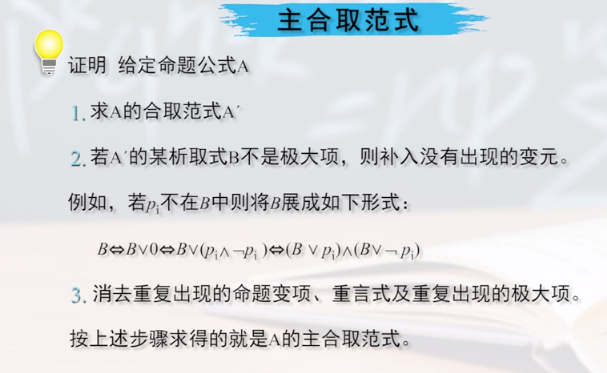## 例题：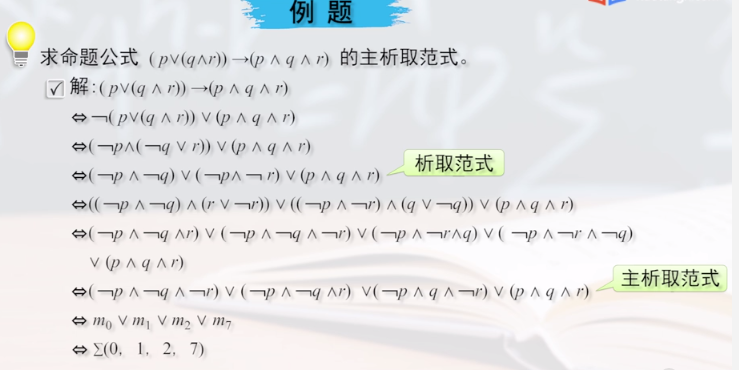这个转化过程看似很麻烦，其实求出析取范式后只需一步便可转化为主析取范式。如下：

析取范式中第一项：（¬ p ∧ \wedge ¬ q） ⇔ \Leftrightarrow m 000 m_{000} ∨ \vee m 001 m_{001} 二进制转十进制为 m 0 m_{0} ∨ \vee m 1 m_{1}
析取范式中第二项：（¬ p ∧ \wedge ¬ r） ⇔ \Leftrightarrow m 000 m_{000} ∨ \vee m 010 m_{010} 二进制转十进制为 m 0 m_{0} ∨ \vee m 2 m_{2}
析取范式中第二项：（ p ∧ \wedge q ∧ \wedge r） ⇔ \Leftrightarrow m 111 m_{111} 二进制转十进制为 m 7 m_{7}

∴ \therefore 主析取范式为 m 0 m_{0} ∨ \vee m 1 m_{1} ∨ \vee m 2 m_{2} ∨ \vee m 7 m_{7} ∑ ( 0 , 1 , 2 , 7 ) \sum (0,1,2,7)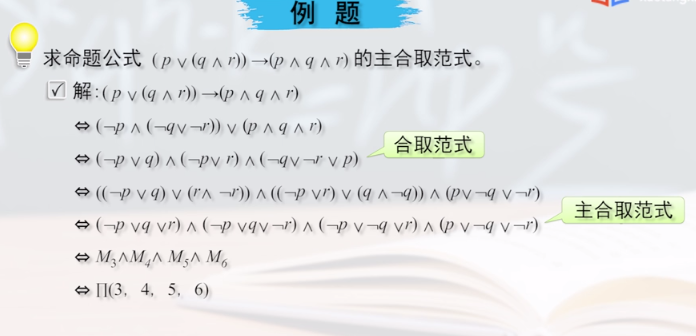同上，这个也可以简便转化，如下：

合取范式中第一项：（¬ p ∨ \vee q） ⇔ \Leftrightarrow M 100 M_{100} ∧ \wedge M 101 M_{101} 二进制转十进制为 M 4 M_{4} ∧ \wedge M 5 M_{5}
合取范式中第二项：（¬ p ∨ \vee r） ⇔ \Leftrightarrow M 100 M_{100} ∧ \wedge M 110 M_{110} 二进制转十进制为 M 4 M_{4} ∧ \wedge M 6 M_{6}
合取范式中第二项：（ p ∨ \vee ¬q ∨ \vee ¬r） ⇔ \Leftrightarrow M 011 M_{011} 二进制转十进制为 M 3 M_{3}

∴ \therefore 主合取范式为 M 3 M_{3} ∧ \wedge M 4 M_{4} ∧ \wedge M 5 M_{5} ∧ \wedge M 6 M_{6} ∏ ( 3 , 4 , 5 , 6 ) \prod (3,4,5,6)

因为我们求m时取的是成真赋值，求M时取得是成假赋值。所以m与M的十进制下标在0 ~ 2 n − 1 2^n-1 这个范围内互补。

## 主析取范式与主合取范式的用途：

1.判断命题公式是否等价

∵ \because 任意命题公式的主析（合）取范式都是存在且唯一的。
∴ \therefore 通过比较他们的主析（合）取范式可以判断两公式是否等价，如下：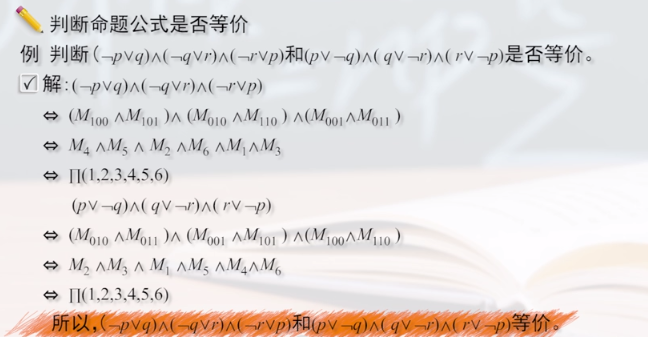2.求公式的成真赋值和成假赋值

这个在上面求m与M的过程中已充分体现，不再赘述。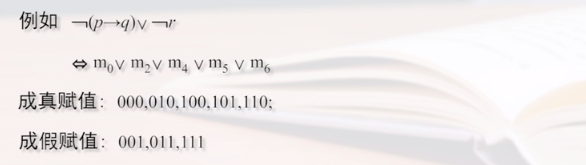3.判断公式类型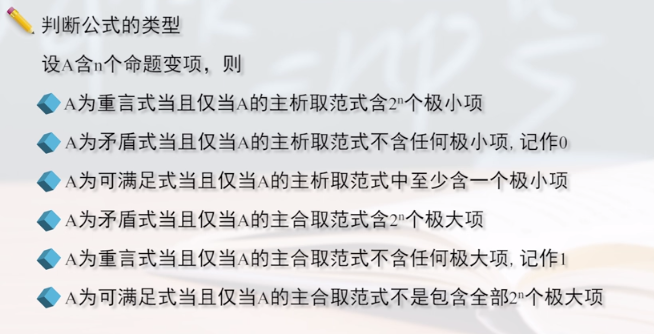例如：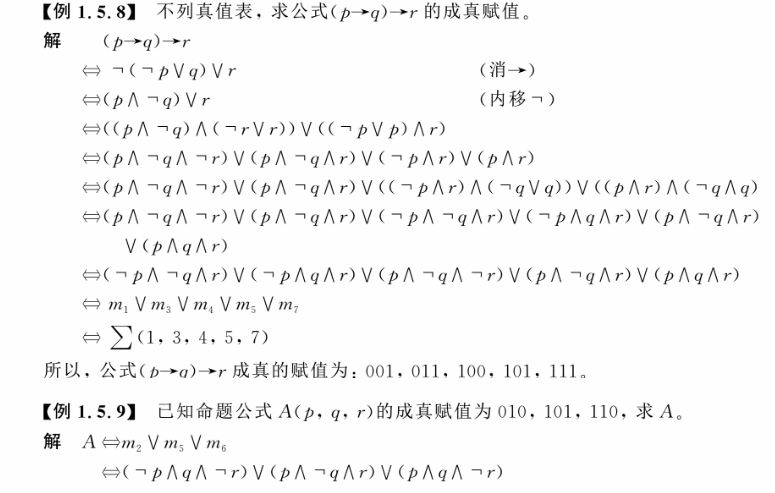4.解决实际问题

还记得我们在等值演算那一小节中的压轴题吗？此类题目也可以用今天讲的东西解，但其实思路都是一样的。都是筛除矛盾的式子，如下：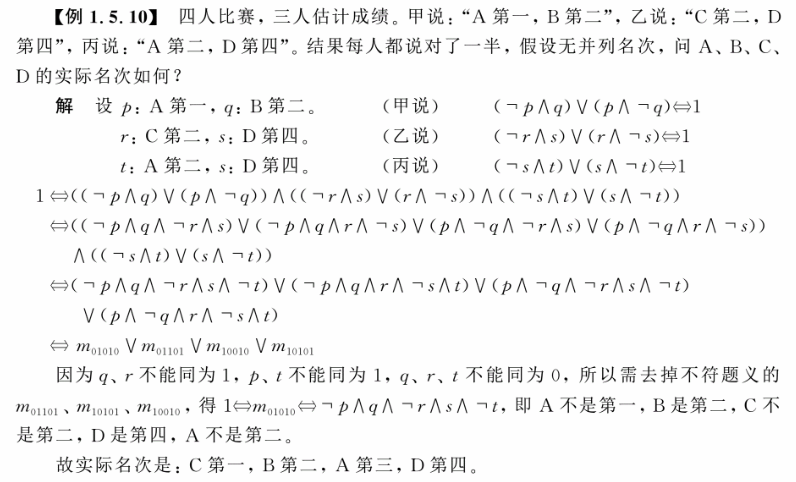## 总结：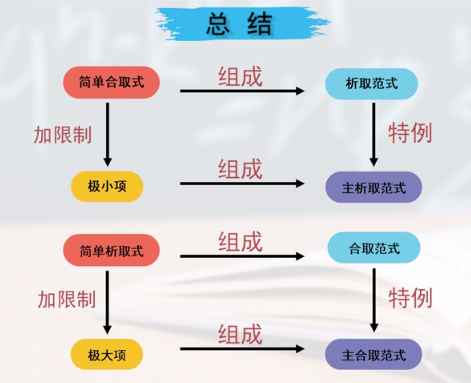## 练习：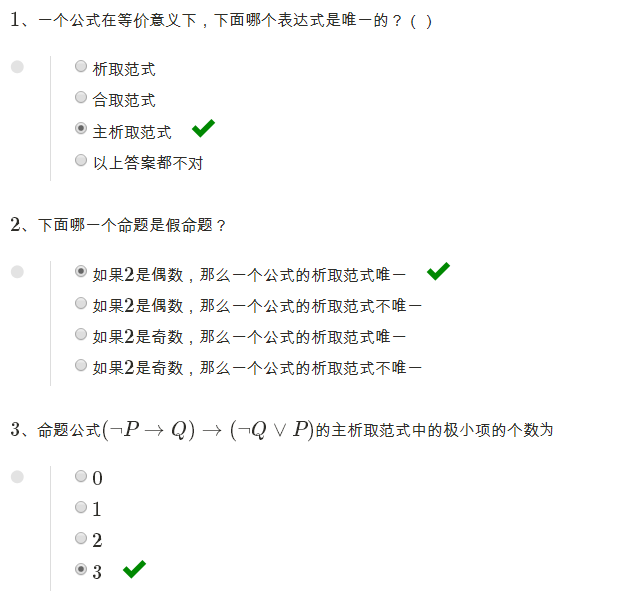展开全文• 研究生人工智能课的作业，主合取范式转主析取范式，敲了四五个小时，亲测可用，python版本的，两个文件，ipynb用anaconda可直接运行，txt的复制到编译器可直接运行，注释详细，需要的自取
• 离散数学析取范式与合取范式PPT学习教案.pptx
• ## 离散数据：析取范式与合取范式

万次阅读 多人点赞 2019-01-09 23:20:21
一、前言 析取范式和合取范式是命题逻辑等值演算中的重要内容，其目的是为了标准化命题公式。下面我将给出析取范式...范式存在定理：任一命题公式都存在之等值的析取范式和合取范式。 求解给定公式范式的步骤：...

一、前言

析取范式和合取范式是命题逻辑等值演算中的重要内容，其目的是为了标准化命题公式。下面我将给出析取范式和合取范式的计算步骤。又由于析取范式和合取范式的形式不唯一，为了便于比较命题公式之间的关系，因此衍生出了主析取范式和主合取范式。所以，也将给出主析取范式和主合取范式的求解方法。

二、范式存在定理

范式存在定理：任一命题公式都存在与之等值的析取范式和合取范式。

求解给定公式范式的步骤：

（1）消去连接词

（2）用双重否定律消去双重否定符，用德摩根律内移否定符

（3）使用分配律：求析取范式时使用的分配律，求合取范式时使用的分配律

三、主析取范式

所有简单合取式都是极小项的析取范式称为主析取范式；

所有简单析取式都是极大项的合取范式称为主合取范式。

表1-1 含p，q的极小项和极大项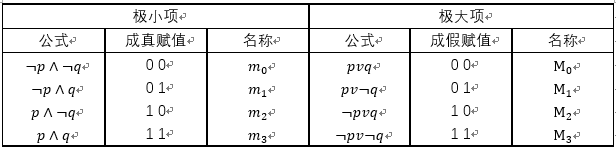展开全文离散数学
• 南京邮电大学实验一真值表法求主析取范式和主合取范式代码 离散数学 实 验 一利用真值表法求取主析取范式以及主合取范式的实现 实验名称：利用真值表法求取主析取范式以及主合取范式的实现 实验目的：通过编程实现主...
• 为了解决粗糙集分辨函数的计算、概念格中内涵缩减的计算、逻辑程序设计的规则简化等问题，抽象出了从合取范式析取范式转换这一核心问题。提出了利用极小覆盖来实现从合取范式析取范式的转换，给出了一个增量式的...
•数学
• 合取范式: 即这个命题表示为子句的合取，每个子句是两个或更多的变量或者它们的非的析取 eg1: 析取范式: 假定用n个命题变量给出一个真值表。证明可依此表构造一个复合命题.使其真值此表一致，构造办法是:对变量...
• 析取范式:若干个极小项的析取.例, 求公式(p∧q)∨r的主析取范式及主合取范式.主析取范式:(p∧q)∨r(p∧q∧(r∨┐r))∨((p∨┐p)∧(q∨┐q)∧r)(p∧q∧r)∨(p∧q∧┐r)∨(p∧q∧r)∨(p∧┐q∧r)∨(┐p∧q∧r)∨(┐p...
• 析取范式 命题公式A如果可等价地写成如下形式： A1 V A2 V … An（n>=1） 其中每个项Ai(i=1,2…,n)是命题变元或其否定形式的合取式，称该公式为A的析取范式。 例：P<->Q <=> (P ^ Q) V (!P ^ !Q) ...
• 通过代码编译出的程序帮助用户求出其输入的命题公式的真值表以及主析取范式和主合取范式。 要求：能够列出含三个以内变量的式公式的真值表，并给出相应的主析取和主合取范式。C++ 离散数学
• 文章目录合取范式（ conjunctive normal form (CNF)）析取范式（disjunctive normal form (DNF)）简化的合取和析取范式标准形式（Canonical form）异或标准形式（xor normal form）二元决策图 form （ROBDD）子句...逻辑学
• 主析取范式与主合取范式 概念性的定义用自己的理解解释，所以有不严谨的地方，以教材为准 范式：是将复杂的复合命题化简成为只有基本联结词的形式（化简掉蕴含，等价） 简单析取（合取）范式：有限个文字构成。 析取...
• 实 验 一利用真值表法求取主析取范式以及主合取范式的实现 实验名称：利用真值表法求取主析取范式以及主合取范式的实现 实验目的：通过编程实现主析取范式以及主合取范式的真值表求法以巩固相关理论的掌握 实验...
• 取范式与合取范式 定义2.2 命题变项及其否定统称作文字。 仅由有限个文字构成的析取式称为简单析取式。 仅由有限个文字构成的合取式称为简单合取式。 例如，文字：p,┐q,r,q. 简单析取式: p,q,p∨q,p∨┐p∨...
• 任意输入一个命题公式，计算并输出其真值表以及主析取范式，主合取范式思路:大概就是将蕴含，等价，异或进行转化,然后使用eval()计算#! /usr/bin/env python3# -*- coding:utf-8 -*-sInput = '' #输入的命题公式字符...
• 析取范式 对任意一个命题公式来说，主析取范式与主合取范式都是唯一的。 命题变元指原子化的，P，Q命题。 极小项的定义：包含全部N个命题变元的合取式，称其为极小项，且N个命题变元中，每个变元与它的否定不能...
• 定理2.3 任何命题公式都存在之等值的主析取范式， 并且是唯一的。 二. 主合取范式 定义： 对于给定的命题公式， 如果有一个等价公式 ，它仅由大项的合取所组成， 则该等价式称为原式的主合取范式。 定理2.4 ...离散数学...